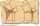# Euclid2

In right triangle ABC with right angle at C is given side a=27 and height v=12. Calculate the perimeter of the triangle.

Result

p =  70.5

#### Solution:Leave us a comment of example and its solution (i.e. if it is still somewhat unclear...):Be the first to comment!#### To solve this example are needed these knowledge from mathematics:

Pythagorean theorem is the base for the right triangle calculator.

## Next similar examples:

1. Euclid 5Calculate the length of remain sides of a right triangle ABC if a = 7 cm and height vc = 5 cm.
2. Euclid3Calculate height and sides of the right triangle, if one leg is a = 81 cm and section of hypotenuse adjacent to the second leg cb = 39 cm.
3. Euclidean distanceCalculate the Euclidean distance between shops A, B and C, where: A 45 0.05 B 60 0.05 C 52 0.09 Wherein the first figure is the weight in grams of bread and second figure is price in USD.
4. Proof PTCan you easy prove Pythagoras theorem using Euclidean theorems? If so, do it.
5. Triangle ABCIn a triangle ABC with the side BC of length 2 cm The middle point of AB. Points L and M split AC side into three equal lines. KLM is isosceles triangle with a right angle at the point K. Determine the lengths of the sides AB, AC triangle ABC.
6. Leg and heightSolve right triangle with height v = 9.6 m and shorter cathetus b = 17.3 m.
7. The ditchDitch with cross section of an isosceles trapezoid with bases 2m 6m are deep 1.5m. How long is the slope of the ditch?
8. Right 24Right isosceles triangle has an altitude x drawn from the right angle to the hypotenuse dividing it into 2 unequal segments. The length of one segment is 5 cm. What is the area of the triangle? Thank you.
9. Vector 7Given vector OA(12,16) and vector OB(4,1). Find vector AB and vector |A|.
10. Isosceles IVIn an isosceles triangle ABC is |AC| = |BC| = 13 and |AB| = 10. Calculate the radius of the inscribed (r) and described (R) circle.
11. ABS CNCalculate the absolute value of complex number -15-29i.
12. Chord - TS v2The radius of circle k measures 87 cm. Chord GH = 22 cm. What is TS?
13. RT and circlesSolve right triangle if the radius of inscribed circle is r=9 and radius of circumscribed circle is R=23.
14. CathetiThe hypotenuse of a right triangle is 41 and the sum of legs is 49. Calculate the length of its legs.
15. Circle annulusThere are 2 concentric circles in the figure. Chord of larger circle 10 cm long is tangent to the smaller circle. What are does annulus have?Given that P = (5, 8) and Q = (6, 9), find the component form and magnitude of vector PQ.We want to prove the sentence: If the natural number n is divisible by six, then n is divisible by three. From what assumption we started?